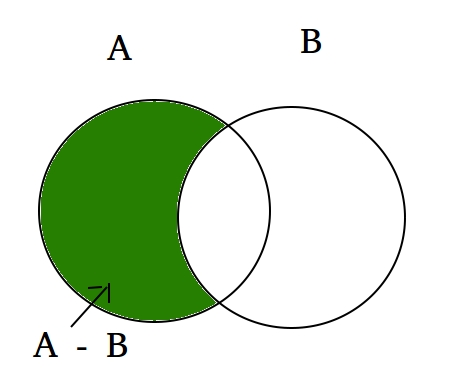Related Articles
Python Set | difference()
• Difficulty Level : Basic
• Last Updated : 31 May, 2020

The difference between the two sets in Python is equal to the difference between the number of elements in two sets. The function difference() returns a set that is the difference between two sets. Let’s try to find out what will be the difference between two sets A and B. Then (set A – set B) will be the elements present in set A but not in B and (set B – set A) will be the elements present in set B but not in set A.
Example:

```set A = {10, 20, 30, 40, 80}
set B = {100, 30, 80, 40, 60}

set A - set B = {10, 20}
set B - set A = {100, 60}

Explanation: A - B is equal to the elements present in A but not in B
B - A is equal to the elements present in B but not in A
```

Let’s look at the Venn diagram of the following difference set function.Syntax:

```set_A.difference(set_B) for (A - B)
set _B.difference(set_A) for (B - A)
```

In this program, we will try to find out the difference between two sets set_A and set_B, both the way:

 `# Python code to get the difference between two sets ` `# using difference() between set A and set B ` ` `  `# Driver Code ` `A ``=` `{``10``, ``20``, ``30``, ``40``, ``80``} ` `B ``=` `{``100``, ``30``, ``80``, ``40``, ``60``} ` `print` `(A.difference(B)) ` `print` `(B.difference(A)) `

Output:

```{10, 20}
{100, 60}
```

We can also use – operator to find the difference between two sets.

 `# Python code to get the difference between two sets ` `# using difference() between set A and set B ` ` `  `# Driver Code ` `A ``=` `{``10``, ``20``, ``30``, ``40``, ``80``} ` `B ``=` `{``100``, ``30``, ``80``, ``40``, ``60``} ` `print` `(A ``-` `B) ` `print` `(B ``-` `A) `

Output:

```{10, 20}
{100, 60}
```

If we have equal sets then it will return the null set.

 `# Python code to get the difference between two sets ` `# using difference() between set A and set B ` ` `  `# Driver Code ` `A ``=` `{``10``, ``20``, ``30``, ``40``, ``80``} ` `B ``=` `{``10``, ``20``, ``30``, ``40``, ``80``, ``100``} ` `print` `(A ``-` `B) `

Output:

```set()
```

Attention geek! Strengthen your foundations with the Python Programming Foundation Course and learn the basics.

To begin with, your interview preparations Enhance your Data Structures concepts with the Python DS Course.

My Personal Notes arrow_drop_up
Recommended Articles
Page :